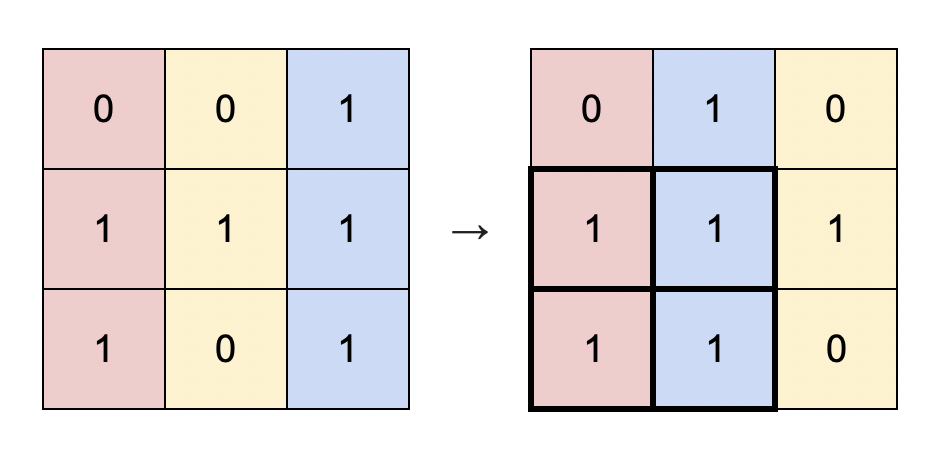# Largest sub matrix rectangle with rearrangement with all 1sTechMunching Jan 17, 2021 · 3 mins read
Share this

We at Tech Munching provide you the cleanest, easy to understand solutions to difficult competitive coding questions. This question #1727 appeared in LeetCode contest #224.Here is the question:

You are given a binary matrix `matrix` of size `m x n`, and you are allowed to rearrange the columns of the `matrix` in any order.

Return the area of the largest submatrix within `matrix` where every element of the submatrix is `1` after reordering the columns optimally.

Example 1:``````Input: matrix = [[0,0,1],[1,1,1],[1,0,1]]
Output: 4
Explanation: You can rearrange the columns as shown above.
The largest submatrix of 1s, in bold, has an area of 4.

``````

Here is our proposed solution:

Not to console the audience reading it after the contest, however, this wasn’t necessarily a `medium` problem. As with life, this problem also has multiple solutions to it. So, please comment down in case you would need further solutions based on DP or so.

Let’s try to look at the problem in a different way, can we think of `value 1` as adding a floor to the building and `value 0` as ground level of the building. Our task is to find the max rectangle with parallel buildings and because the columns can be rearranged, we can move the buildings.

Here are the height of the building for each row:

Col 1 Col 2 Col 3
Row 1 0 0 1
Row 2 1 1 2 (Previous floor adds up in coloumn 3)
Row 3 2 0 3 (Ground floor in coloumn 2 nullifies previous height)Since, we can actually move the coloumns, above rows after sorting can be represented as:

Col 1 Col 2 Col 3
Row 1 1 0 0
Row 2 2 1 1
Row 3 3 2 0Once you reach this state, finding the max rectangles for each building is height * width.

For example:

Row 3 & Col 1 : 3 (height) * 1 (width)

Row 3 & Col 1 & Col 2 : 2 (height) * 2 (width)

Here is complete solution:

``````class Solution {
public:
int largestSubmatrix(vector<vector<int>>& matrix) {
if (matrix.empty()) return 0;

vector<int> brickLevel (matrix.size(), 0);

int answer = numeric_limits<int>::min();

for (auto i = 0; i < matrix.size(); i++) {
for (auto j = 0; j < matrix.size(); j++) // set the brick value
brickLevel[j] = matrix[i][j] == 0 ? 0 : brickLevel[j] + 1;

// sort bricks to keep buildings consecutive
auto mx = brickLevel;
sort(mx.begin(), mx.end(), greater());

for (auto i = 0; i < mx.size(); i++)
answer = max(answer, (i + 1) * mx[i]);
}
return answer;
}
};
``````

The time complexity for a matrix of `MxN` will be `MNlog(N)`. That’s easy to understand, the outer loop iterates M rows which iterates N columns and sort vector of length N which is `M * (N + Nlog(N))`, which asymptotically is `MNlog(N)`.

Please feel free to comment down below in case you find any flaw or would need deeper understanding in any aspects.

##### Join Newsletter
Get the latest news right in your inbox. We never spam!##### Written by TechMunching
Hi, We are Tech Munching. We hope you love it!